# CIE A Level Maths: Pure 3复习笔记8.1.3 Square Roots of a Complex Number

### Square Roots of a Complex Number

#### How do I find the square root of a complex number?

• The square roots of a complex number will themselves be complex:
• i.e. if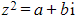then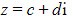• We can then square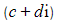and equate it to the original complex number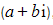, as they both describe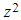:
•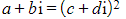• Then expand and simplify:
•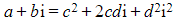•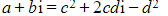• As both sides are equal we are able to equate real and imaginary parts:
• Equating the real components: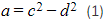• Equating the imaginary components: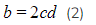• These equations can then be solved simultaneously to find the real and imaginary components of the square root
• In general, we can rearrange (2) to make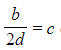and then substitute into (1)
• This will lead to a quartic equation in terms of d; which can be solved by making a substitution to turn it into a quadratic (see 1.1.5 Further Solving Quadratic Equations (Hidden Quadratics))
• The values of d can then be used to find the corresponding values of c, so we now have both components of both square roots (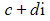)
• Note that one root will be the negative of the other root
• g.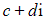and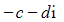#### Worked Example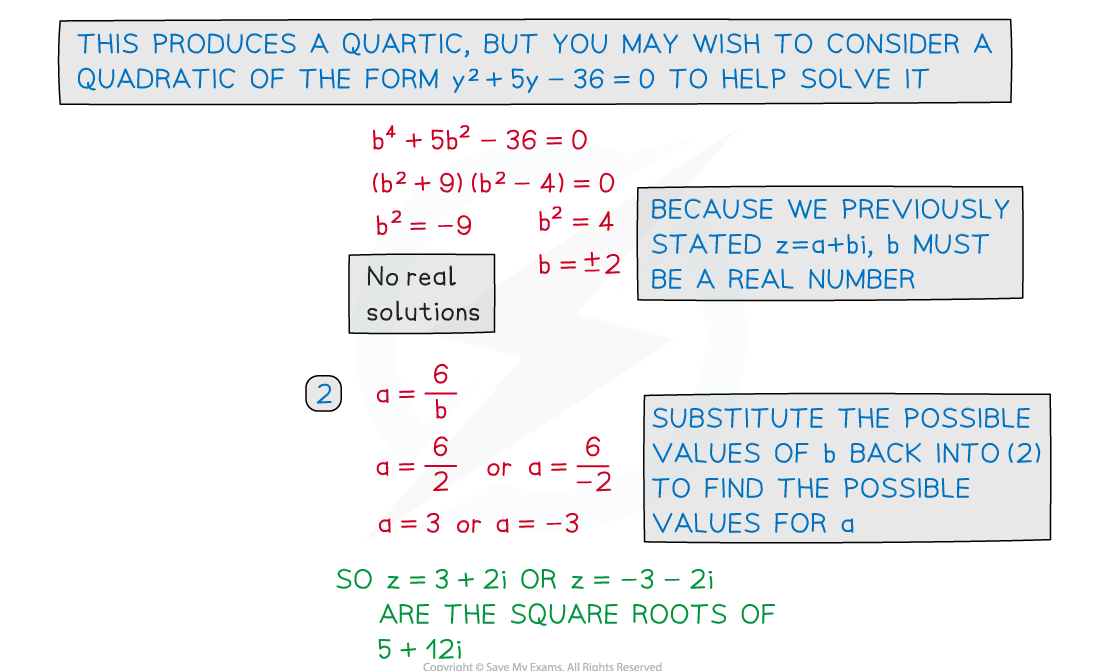#### Exam Tip

• Most calculators used at A-Level can handle complex numbers.
• Once you have found the square roots algebraically; use your calculator to square them and make sure you get the number you were originally trying to square-root!